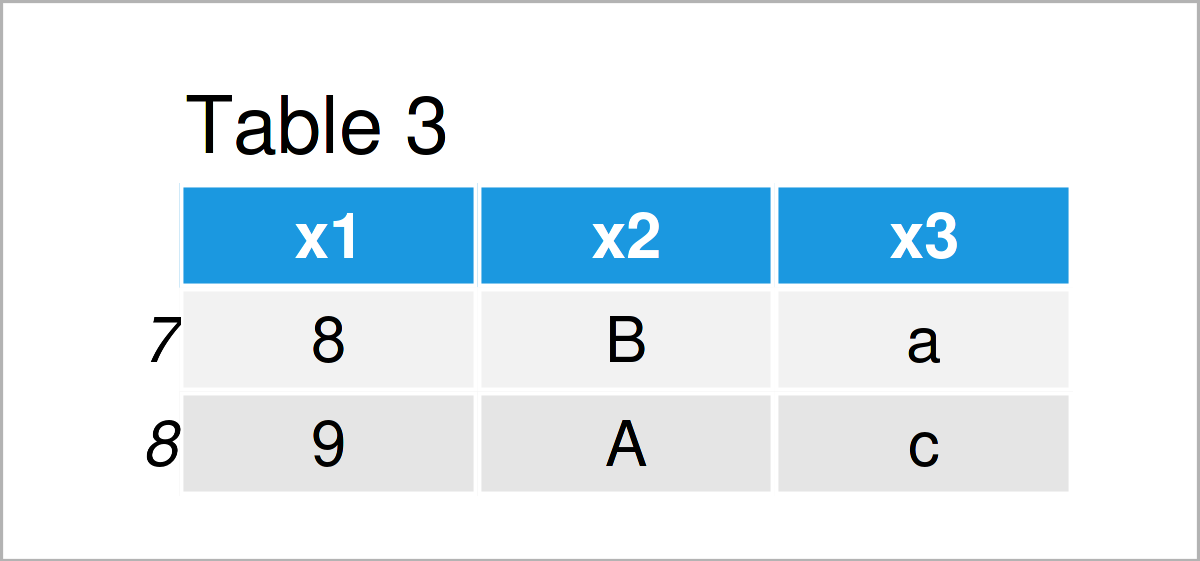# Extract Top & Bottom N Rows from pandas DataFrame in Python (2 Examples)

On this page you’ll learn how to select the top and bottom N rows of a pandas DataFrame in Python.

You’re here for the answer, so let’s get straight to the examples…

## Example Data & Libraries

First, we need to load the pandas library:

`import pandas as pd # Load pandas library`

We’ll also have to create some example data:

```data = pd.DataFrame({'x1':range(1, 10), # Create example DataFrame 'x2':['C', 'A', 'B', 'B', 'A', 'C', 'A', 'B', 'A'], 'x3':['a', 'b', 'd', 'b', 'c', 'b', 'd', 'a', 'c']}) print(data) # Print example DataFrame```As you can see based on Table 1, our example data is a DataFrame containing nine rows and three columns called “x1”, “x2”, and “x3”.

The following syntax explains how to select only the first few rows of a pandas DataFrame using the Python programming language.

For this task, we can apply the head function. Within the head function, we have to specify the number of rows that we want to extract from our data set.

Have a look at the following Python syntax:

```data_head = data.head(4) # Apply head function print(data_head) # Print head of DataFrame```After running the previous Python programming code a new pandas DataFrame called data_head shown in Table 2 has been created.

As you can see, this new data matrix contains only the first four lines of our original DataFrame.

## Example 2: Return Bottom N Rows of pandas DataFrame Using tail() Function

Example 2 explains how to keep only the bottom part of a pandas DataFrame.

Similar to Example 1, we can use the tail function to do this. Note that we are specifying the value 2 instead of the values 4 in this example.

```data_tail = data.tail(2) # Apply tail function print(data_tail) # Print tail of DataFrame```The output of the previous Python code is shown in Table 3: We have created another pandas DataFrame containing the last two rows of our input DataFrame.

## Video, Further Resources & Summary

Would you like to know more about the extraction of the top and bottom N rows of a pandas DataFrame and similar topics to this? Then I recommend watching the following video on the Data School YouTube channel.

In the video instruction, the speaker explains how to filter and extract certain rows from a pandas DataFrame using the Python programming language – a similar topic to the content of the tutorial shown on this page:

Please accept YouTube cookies to play this video. By accepting you will be accessing content from YouTube, a service provided by an external third party.If you accept this notice, your choice will be saved and the page will refresh.

In addition, you may read some of the other Python tutorials on my website.